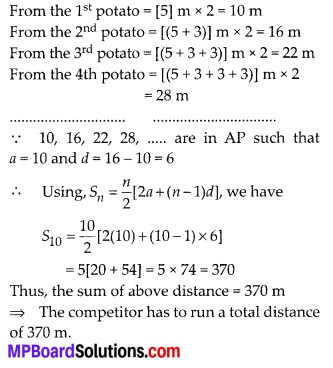In this article, we will share MP Board Class 10th Maths Book Solutions Chapter 5 Arithmetic Progressions Ex 5.3 Pdf, These solutions are solved subject experts from the latest edition books.

## MP Board Class 10th Maths Solutions Chapter 5 Arithmetic Progressions Ex 5.3Question 1.
Find the sum of the following APs:
(i) 2,7,12, ….. to 10 terms
(ii) -37, -33, -29, …. to 12 terms
(iii) 0.6, 1.7, 2.8, …. to 100 terms
(iv) $$\frac{1}{15}, \frac{1}{12}, \frac{1}{10}$$, ……
Solution:
(i) Here, a = 2, d = 7 – 2 = 5, n = 10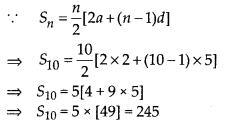Thus, the sum of first 10 terms is 245.

(ii) Here, a = -37, d = -33 – (-37) = 4, n = 12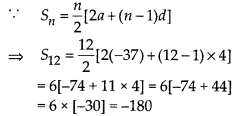Thus, the sum of first 12 terms is -180.

(iii) Here, a = 0.6, d = 1.7 – 0.6 = 1.1, n = 100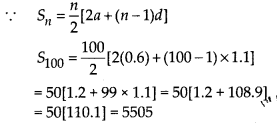Thus, the required sum of first 100 terms is 5505.

(iv)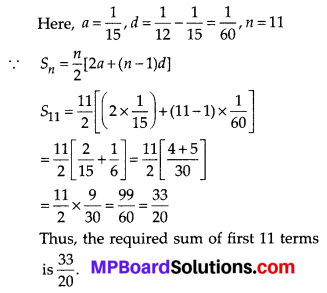Thus, the required sum of first 11 terms is $$\frac{33}{20}$$Question 2.
Find the sums given below:
(i) 7+10$$\frac{1}{2}$$ + 14 + …… + 84
(ii) 34 + 32 + 30+ …….. + 10
(iii) -5 + (-8) + (-11) + ……. + (-230)
Solution:
(i) Here,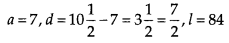Let n be the number of terms.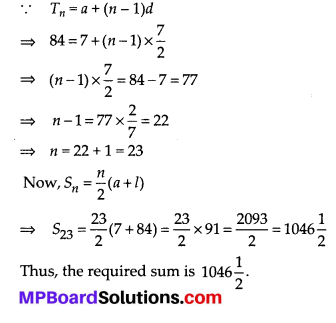(ii) Here, a = 34, d = 32 – 34 = -2, l = 10
Let n be the number of terms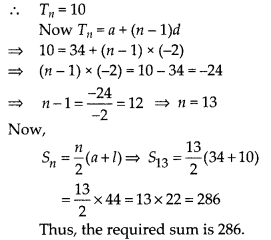(iii) Here, a = -5,d = -8- (-5) = -3,1 = -230
Let n be the number of terms.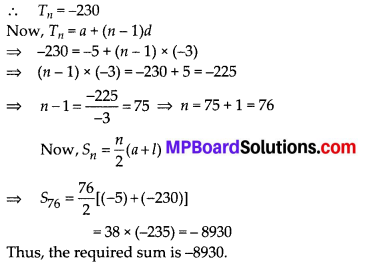Question 3.
In an AP:
(i) given a = 5, d = 3, an = 50,find n and Sn.
(ii) given a = 7, a13 = 35, find d and S13.
(iii) given a12 = 37, d = 3, find a and S12.
(iv) given a3 = 15, S10 = 125, find d and a10.
(v) given d = 5, S9 = 75, find a and a9.
(vi) given a = 2, d = 8, Sn = 90, find n and an.
(vii) given a = 8, an = 62, Sn = 210, find n and d.
(viii) given an = 4, d = 2,Sn = -14, find n and a.
(ix) given a = 3, n = 8, S = 192, find d.
(x) given l = 28, S = 144, and there are total 9 terms. Find a.
Solution:
(i) Here, a = 5, d = 3 and an = 50 = l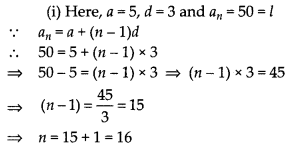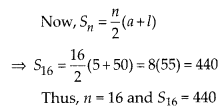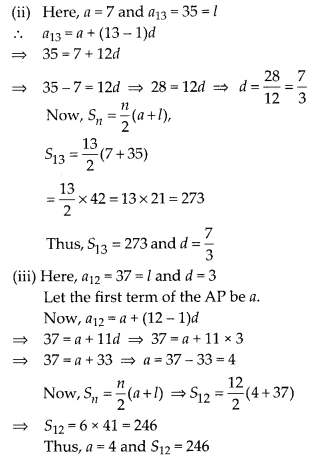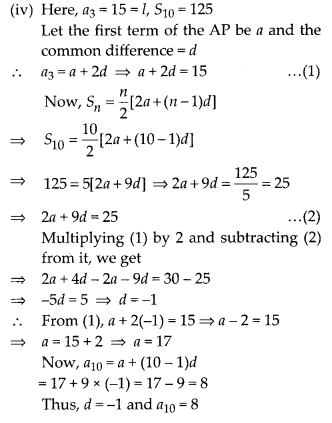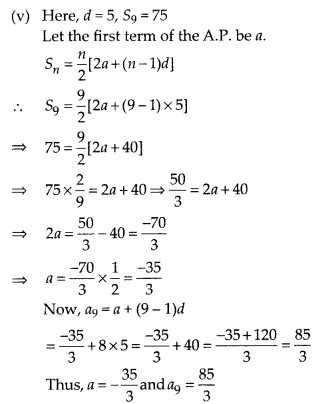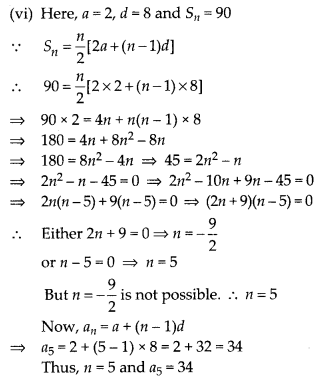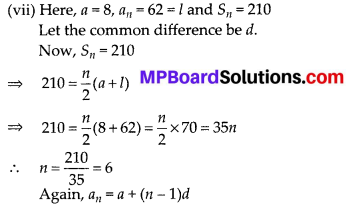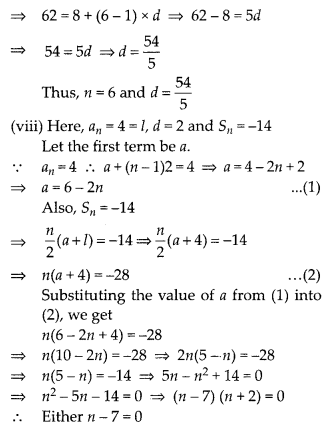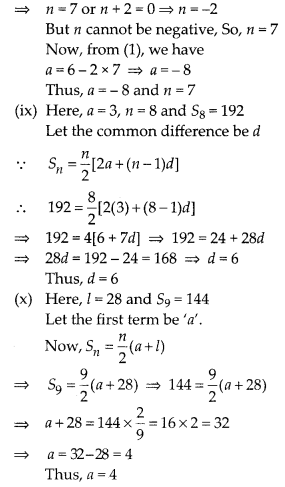Question 4.
How many terms of the AP : 9,17, 25, ….. must be taken to give a sum of 636?
Solution:
Here, a = 9, d = 17 – 9 = 8, Sn = 636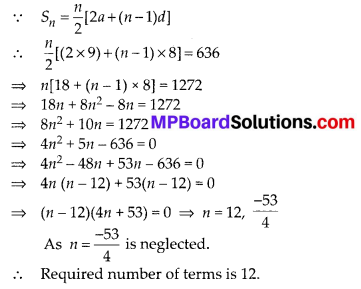Question 5.
The first term of an AP is 5, the last term is 45 and the sum is 400. Find the number of terms and the common difference.
Solution: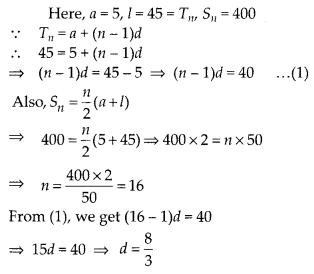Question 6.
The first and the last terms of an A.P. are 17 and 350 respectively. If the common difference is 9, how many terms are there and what is their sum?
Solution:
We have, first term (a) = 17, last term (l) = 350 = Tn and common difference (d) = 9
Let the number of terms be n.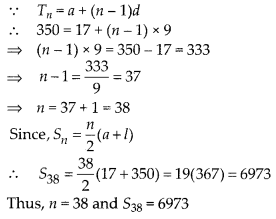Question 7.
Find the sum of first 22 terms of an AP in which d = 7 and 22nd term is 149.
Solution:
Here, n = 22, T22 = 149 = l, d = 7
Let the first term of the AP be a.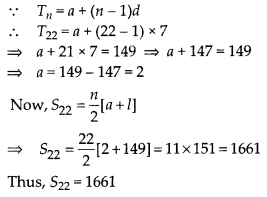Question 8.
Find the sum of first 51 terms of an AP whose second and third terms are 14 and 18 respectively.
Solution: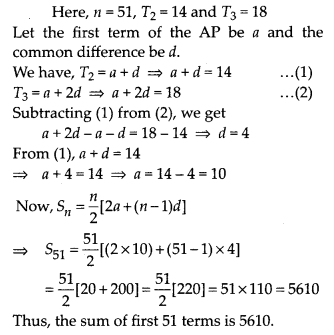Question 9.
If the sum of first 7 terms of an AP is 49 and that of 17 terms is 289, find the sum of first n terms.
Solution:
Here, we have S7 = 49 and S17 = 289
Let the first term of the AP be a and d be the common difference, then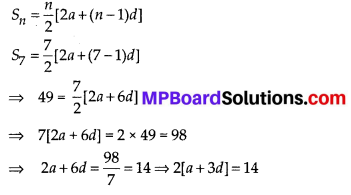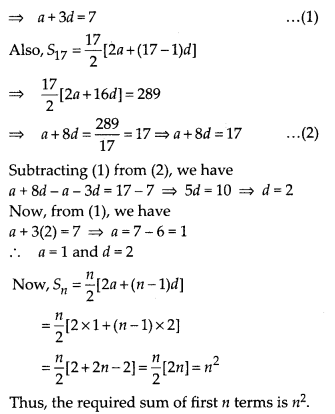Question 10.
Show that a1, a2,…… an,………, form an AP where an is defined as below:
(i) an = 3 + 4n
(ii) an = 9 – 5n
Also find the sum of the first 15 terms in each case.
Solution: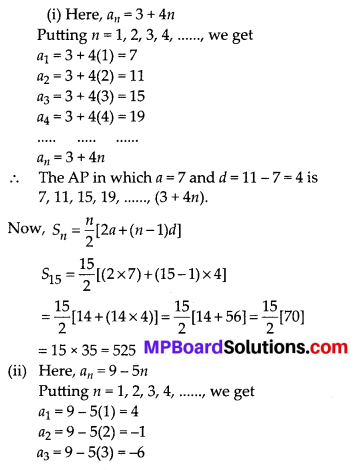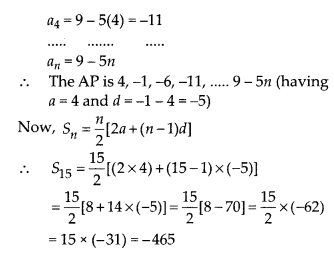Question 11.
If the sum of the first n terms of an AP is 4n – n2, what is the first term (that is S1)? What is the sum of first two terms? What is the second term? Similarly, find the 3rd, the 10th and the nth terms.
Solution:
We have, Sn = 4n – n2
S1 = 4(1) – (1)2 = 4 – 1 = 3 ⇒ First term (a) = 3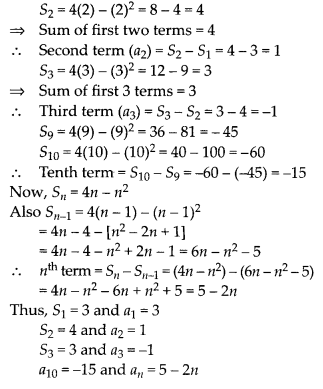Question 12.
Find the sum of the first 40 positive integers divisible by 6.
Solution:
The first 40 positive integers divisible by 6 are 6,12,18, ……. , (6 × 40)
And, these numbers are in AP, such that a = 6,
d = 12 – 6 = 6 and n = 6 × 40 = 240 = l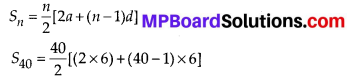= 20[12 + 39 × 6] = 20[12 + 234]
= 20 × 246 = 4920

Question 13.
Find the sum of the first 15 multiples of 8.
Solution:
The first 15 multiples of 8 are 8, 16, 24, 32, ….., 120.
These numbers are in AP, where, a = 8 and l = 120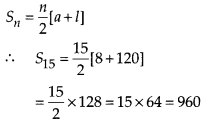Thus, the sum of first 15 multiples of 8 is 960.Question 14.
Find the sum of the odd numbers between 0 and 50.
Solution:
Odd numbers between 0 and 50 are 1, 3, 5, 7, …… , 49
These numbers are in AP such that a = 1 and l = 49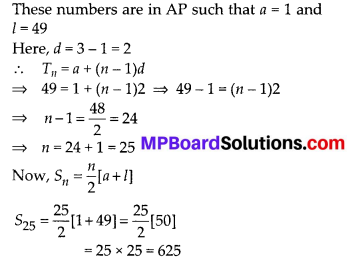Thus, the sum of odd numbers between 0 and 50 is 625.

Question 15.
A contract on construction job specifies a penalty for delay of completion beyond a certain date as follows : ₹ 200 for the first day, ₹ 250 for the second day, ₹ 300 for the third day, etc., the penalty for each succeeding day being ₹ 50 more than for the preceding day. How much money the contractor has to pay as penalty, if he has delayed the work by 30 days?
Solution:
Here, penalty for delay on 1st day = ₹ 200
2nd day = ₹ 250
3rd day = ₹ 300
……………………………………….
……………………………………….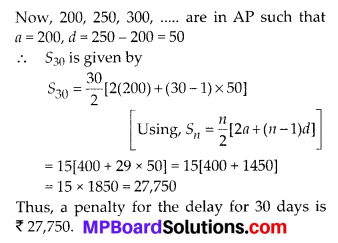Question 16.
A sum of ₹ 700 is to be used to give seven cash prizes to students of a school for their overall academic performance. If each prize is ₹ 20 less than its preceding prize, find the value of each of the prizes.
Solution:
Sum of all the prizes = ₹ 700
Let the first prize be a
2nd prize = (a – 20)
3rd prize = (a- 40)
4th prize = (a – 60)
The above prizes form an AP
Now, we have, first term = a
Common difference = d = (a – 20) – a = -20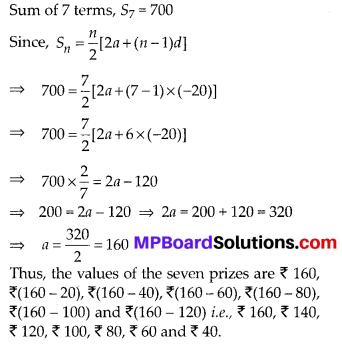Question 17.
In a school, students thought of planting trees in and around the school to reduce air pollution. It was decided that the number of trees, that each section of each class will plant, will be the same as the class, in which they are studying, e.g., a section of Class I will plant 1 tree, a section of Class II will plant 2 trees and so on till Class XII. There are three sections of each class. How many trees will be planted by the students ?
Solution:
Number of classes = 12
∵ Each class has 3 sections.
∴ Number of trees planted by class I = 1 × 3 = 3
Number of trees planted by class II =2 × 3 = 6
Number of trees planted by class III = 3 × 3 = 9
Number of trees planted by class IV = 4 × 3 = 12
………………………………………………………………….
Number of trees planted by class XII = 12 × 3 = 36
The numbers 3, 6, 9,12, ……. , 36 form an AP
Here, a = 3, d = 6 – 3 = 3 and n = 12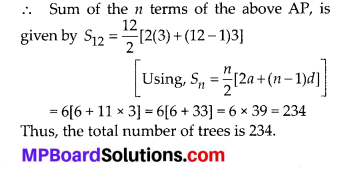Question 18.
A spiral is made up of successive semi-circles, with centres alternately at A and B, starting with centre at A, of radii 0.5 cm, 1.0 cm, 1.5 cm, 2.0 cm, …… as shown in figure. What is the total length of such a spiral made up of thirteen consecutive semi-circles? (Take $$\pi=\frac{22}{7}$$)Solution: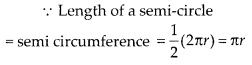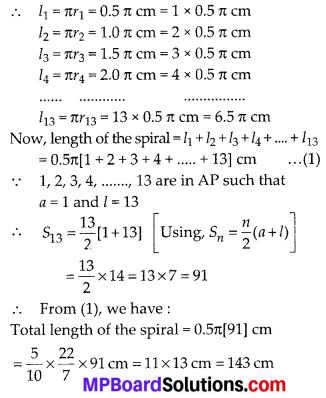Question 19.
200 logs are stacked in the following manner: 20 logs in the bottom row, 19 in the next row, 18 in the row next to it and so on (see figure). In how many rows are the 200 logs placed and how many logs are in the top row?Solution: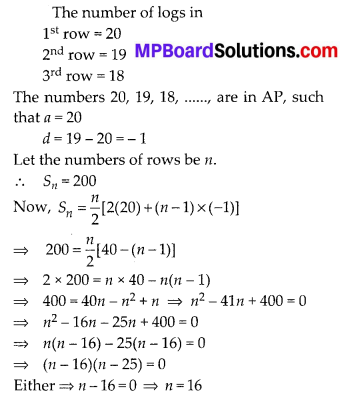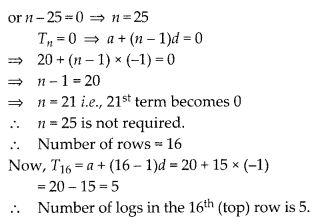Question 20.
In a potato race, a bucket is placed at the starting point, which is 5 m from the first potato, and the other potatoes are placed 3 m apart in a straight line. There are ten potatoes in the line (see figure).A competitor starts from the bucket, picks up the nearest potato, runs back with it, drops it in the bucket, runs back to pick up the next potato, runs to the bucket to drop it in, and she continues in the same way until all the potatoes are in the bucket. What is the total distance the competitor has to run?
[Hint :To pick up the first potato and the second potato, the total distance (in metres) run by a competitor is 2 × 5 + 2 × (5 + 3)]
Solution:
Here, number of potatoes = 10
The to and fro distance of the bucket: